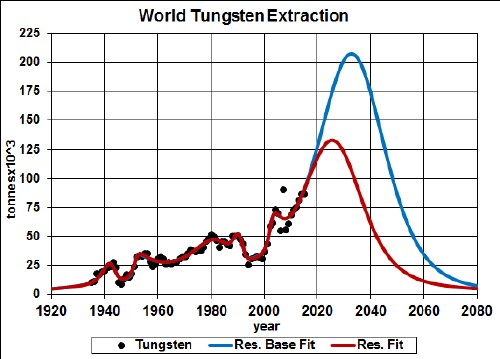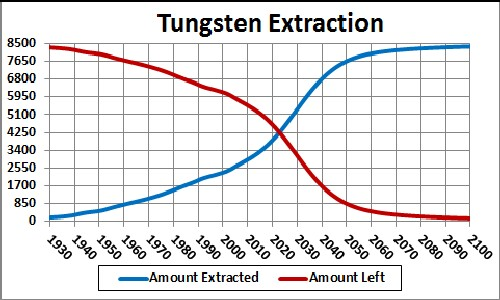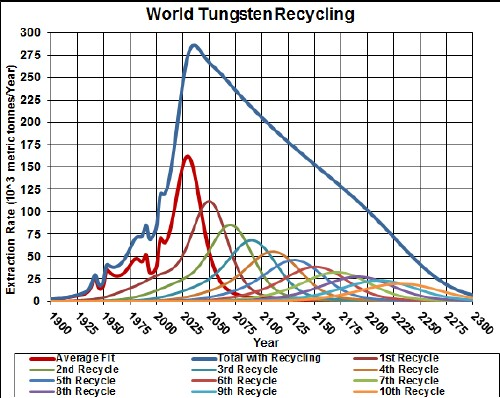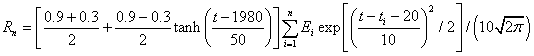# Tungsten Depletion Including Recycling

Tungsten is used to make high-strength steel alloys. The graph below shows the tungsten extraction data for the world and two Verhulst function fits to the data in order to extrapolate into the future.Tungsten extraction rate for the world and Verhulst function fits to the data.

The red curve is a fit with total amount to be extracted that incorporates the latest reserves estimate (~35 x 106 tonnes), and the blue curve is fit that incorporates the latest reserves-base estimate (~63 x 106 tonnes),

It appears that world-tungsten extraction will peak before 2035.

Taking an average extraction curve of the two fits, the crossover point at ~2023 when the amount extracted is equal to the amount left to be extracted is shown here:## Recycling

tungsten, especially the tungsten used in batteries, is recycled to a high degree. Assume that:

• The tungsten-extraction curve is the average of the two curves given above.
• Recycling of tungsten follows a hyperbolic tangent curve from 30% to 90% recycling with a break point of year 1980 and width 50 years.
• The recycling is delayed by a Gaussian curve peaking at a delay of 20 years and a width of 10 years.

The effective tungsten available for making items after the first ten recycling cycles is shown in the following graph, along with the effective tungsten available for each cycle:The equation for a recyling cycle is,

where Ei is the amount available from the previous cycle. Here is an example of the Excel coding:

{=((\$J\$2+\$I\$2)/2+((\$J\$2-\$I\$2)/2)*TANH((A27-\$K\$2)/\$L\$2))*SUM(\$I\$27:I27*(EXP(-1*((A27-\$A\$27:A27-\$N\$2)/\$O\$2)^2/2))/\$O\$2/SQRT(2*PI()))} (The curly bracket surrounding the term makes it into an array; it must be entered by holding down the SHIFT & CTRL keys while pressing the ENTER key.)

Of course, the recycling could be extended to more cycles, skewing the curve further into the future. However, the peak and fall off after it will not change because further cycles are essentally zero in that time region.

Thus, under the assumptions given above, the effective amount of tungsten available for making items peaks at about 2100 and falls off rapidly after that. Humans will have taken concentrated tungsten deposits and scattered them across the surface of the earth.

The Excel spreadsheet is set up to make it easy to calculate with different recycling assumptions.

Minerals Depletion